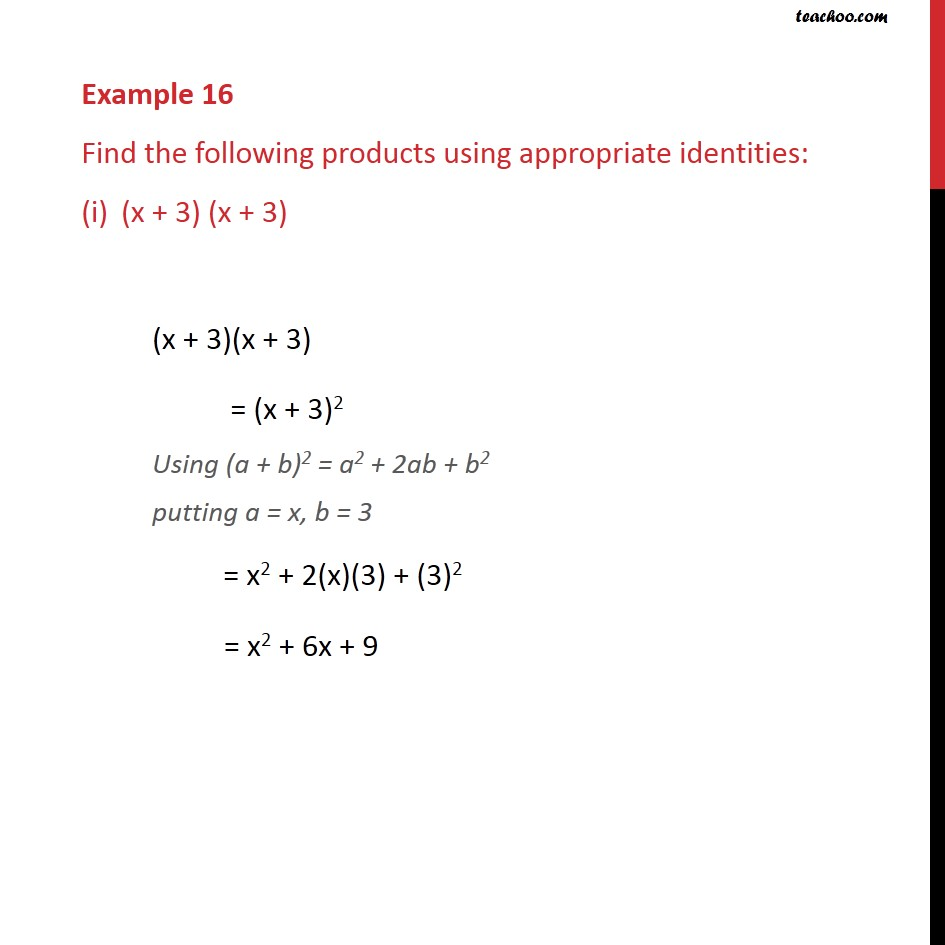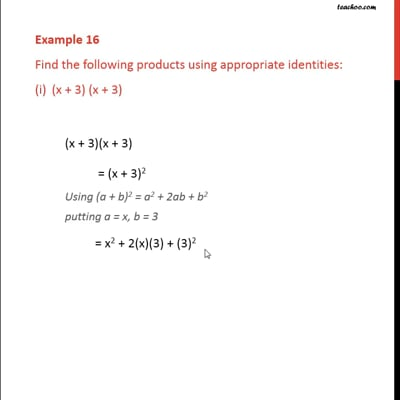Examples

Chapter 2 Class 9 Polynomials
Serial order wiseThis video is only available for Teachoo black users

Maths Crash Course - Live lectures + all videos + Real time Doubt solving!

### Transcript

Example 16 Find the following products using appropriate identities: (x + 3) (x + 3) (x + 3)(x + 3) = (x + 3)2 Using (a + b)2 = a2 + 2ab + b2 putting a = x, b = 3 = x2 + 2(x)(3) + (3)2 = x2 + 6x + 9